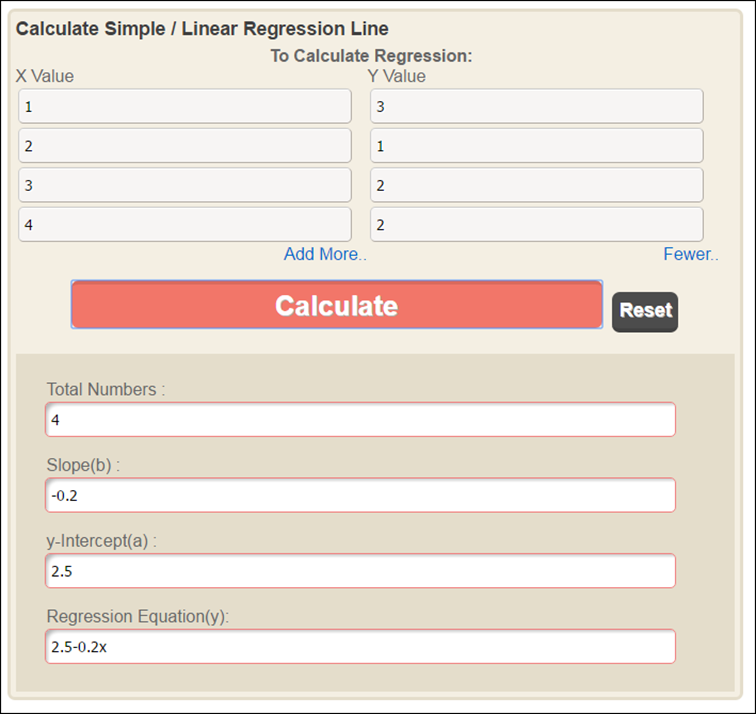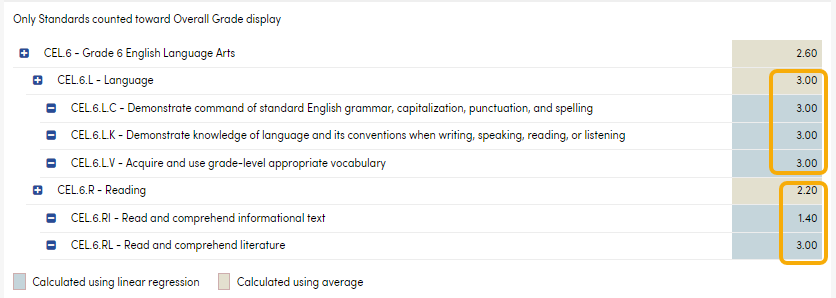The gradebook calculations for a rubric, standards-based gradebook is different than the point-based or assignment based gradebook. Below is general information on how the gradebook overall score is calculated in rubric standard-based gradebooks:

• Only Summative assignments are used in the calculation. Formative are not used in the grade calculation.
• The overall score calculates by standard, so linear regression is used to calculate the score for the assignments with the same standard.
• Assignments that are at the same level will have their trended scores averaged (NOTE: the trended value is bound by the lowest score and highest score the student received, so the trended grade can never be below or above a grade the student already achieved).
• If there is only one assignment tied to a standard, then that assignment’s score is used as is (no trending or averaging because there is nothing to trend or average with).
• If there are only two assignments tied to a standard, then the last assignment’s score is used as the score for that standard (again, no trending is done to calculate the score for that standard).
• If there are 3 or more assignments tied to a standard, then the scores are trended to find the overall score for that standard.
• Work from the bottom – up (i.e. from the lowest level most granular standard all the way up)
• The Gradebook Detail page (Student Data > Grades >Gradebook Detail) shows a breakdown on how the grades are calculated. Select the rubric gradebook, click on standards, open up the assignment/standards tree completely and work from the bottom up.
• The Standards Based Progress Dashboard ( Student Data > Grades > Standards Based Progress Dashboard ) also shows a breakdown of the grades in both graphical form and in detail. Click on View Related to drill down to view the charts for the lower level standards. Click on View Details to list the assignment scores that apply to that standard (again, work from the bottom up to see how the assignment scores affect the grade for that standard). Please see Standards Based Progress Dashboard for more information about this feature.
• This site can be used to produce the linear regression equation to determine the trended grade for a standard: https://www.easycalculation.com/statistics/regression.php

### How to Use the Linear Regression Calculator to Verify the Trended Grade for Each Standard:

The X is the assignment number, the Y is the score for the assignment…

In this example the scores for the first 4 assignments are entered (3,1,2,2), the 5th assignment score will be the overall score for the standard (we use the “next” score as the calculated overall score for the standard):Using the equation provided from the site to calculate what the next grade is trending towards by substituting the number of the next assignment (in this example 5) for the x value:

2.5 – 0.2(x) =

2.5 – 0.2(5) =

1.5

NOTE:  The trended score will be within the minimum and maximum values of all scores in the calculation, so since this set of scores went from 1 to 3 the trended score will be >=1 and <=3
(i.e., students will not have a trended score below the minimum or above the maximum of all scores received for that standard)

Select the rubric standards-based gradebook from the dropdown in the blue header.

Open up all of the standards to the assignment level.Use the assignment grades for each standard in the Linear Regression formula to verify the trended grade for each standard (blue boxes in the below example).Once all of the lowest level standards have been verified close the view to the assignments to remove the visual clutter. Standards that are at the same level are averaged to determine the rubric score of the next higher standard. (see brown boxes).Continue closing the verified standard levels and working up to the highest level standard. In this example the Language and Reading standards are at the same level and their rubric grades are averaged to determine the overall English Language Arts grade.